# Monetary policy in the CNB's macroeconomic forecast

The forecast is the key source material for the CNB Bank Board's decisions. The forecast captures the functioning of the entire economy, including monetary policy (see the July 2002 Inflation Report). 1 The model on which the forecast is based contains a simple central bank reaction function which is in line with the definition of the CNB's objectives and approximately expresses the past interest rate path. The output of the forecast is the implied interest rate trajectory.

However, this implied trajectory cannot be viewed as binding as regards the future path of interest rates. The arrival of new information following publication of the forecast can change the outlook for the evolution of the economy and the related consistent reaction of the central bank. The second reason is the fact that no simple, model reaction function can entirely precisely express the detailed monetary-policy debate that precedes the decision on the interest rate setting.

The specification of the reaction function used in the CNB's forecasting system assumes that nominal interest rates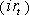are changed in response to an expected deviation of inflation from the inflation target in four quarters' time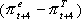andthe present output gap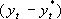. However, given the considerable uncertainties surrounding monetary policy, interest rates are not changed at once, but gradually, in several steps. This is expressed in the reaction function specification with a lagged interest rate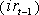.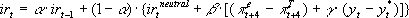If the economy is in equilibrium, i.e. if it has not been disturbed from equilibrium by any demand or supply shocks, interest rates are equal to the "policy neutral" interest rates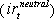, given by equilibrium real interest rates and forecast inflation.

The response of interest rates to economic developments is characterised approximately by the individual parameters of the reaction function (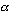,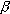,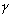). The degree of uncertainty and the distribution of the change in interest rates into several steps are expressed by the smoothing coefficient (). The aggression coefficient () determines the strength of the reaction of interest rates to the expected deviation of inflation from the target and the output gap. Coefficientdetermines the ratio of the deviation of expected inflation from the target to the output gap. In line with the CNB's defined objectives, this ratio is set strongly in favour of inflation. The specific values of each parameter undergo gradual changes over time depending on how the data base used for their estimation expands.